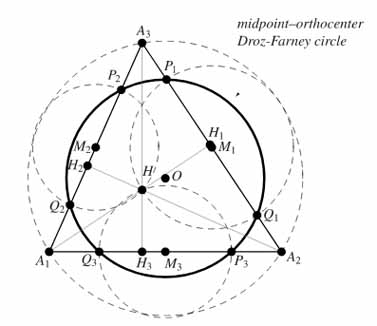# Droz-Farny Circle TheoremIf equal circles are drawn on the vertices of a triangle they cut the lines joining the midpoints of the triangle in six points. These six points lie on a circle whose centre is the orthocentre of the triangle.

Diagram from mathworld.wolfram.com.